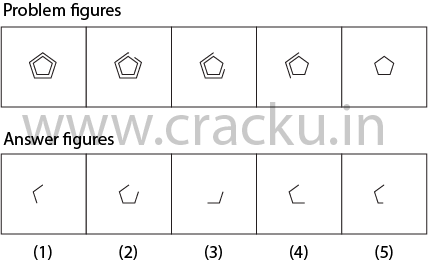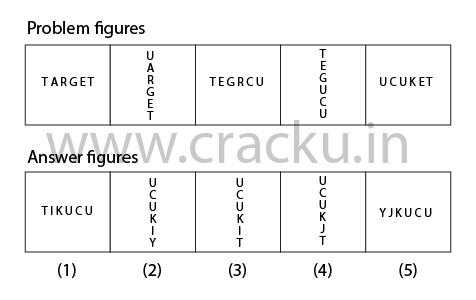## Figures

Theory

Reasoning figures are often the most challenging questions in the IBPS exam. They constitute upto 20% of the reasoning questions. Figures can be designed using words, numbers and shapes or combinations of them. They require the candidate to select the next figure in the sequence from the given options. Given the time constraint in the exam, it may be a good idea to attempt these questions at the end, after the less time consuming ones.

Tip

The approach is to identify if the patterns in the figures repeat themselves in 2, 3 or 4 figures. The pattern may involve 2 figures ie similar changes happen from figure 3 to figure 4 as they do from figure 1 to figure 2.
1 => 2
3 =>4
In 3 figure patterns, the change from 1 to 2 and from 2 to 3 is similar to the change from 4 to 5 and from 5 to the answer figure.
1 => 2 => 3
Process of elimination can be very effective in shortlisting the options.

Solved ExampleSolution:
The pentagon loses half a side then one and a half then three and then five sides.
It follows a pattern of .5, 1.5, 3, 5, ?
The difference between the numbers increases by .5
1.5 - .5 = 1
3 - 1.5 = 1.5
5 - 3 = 2
? - 5 = 2.5
So, ? = 7.5
So, the pentagons lose 7.5 sides in the answer figure.

Solved ExampleSolution:
In every figure, the letters rotate 90 degrees. In every figure, the n-1 th letter is replaced by the n-1th letter to the right in the English Alphabetical Series.
For e.g., in the second figure, (n =2) T is TARGET is replaced by U. Then A is replaced by C, etc.
In the final figure, ie 6th figure, the fifth letter ie E is replaced by J which is 5 places to the right in the alphabetical series.